## How to Calculate and Solve for Steady State Diffusion | Diffusion in Alloying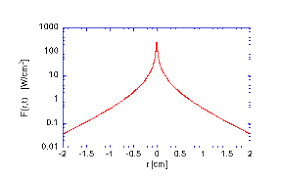The image above represents steady state diffusion.

To compute for steady state diffusion, three essential parameters are needed and these parameters are Diffusion Gradient (dC/dx), Cross-sectional Area (A) and Time (t).

The formula for calculating steady state diffusion:

J = dC/dx/At

Where:

A = Cross-sectional Area
t = Time

Given an example;
Find the steady state diffusion when the diffusion coefficient is 12, the cross-sectional area is 4 and time is 2.

This implies that;

dC/dx = Diffusion Gradient = 12
A = Cross-sectional Area = 4
t = Time = 2

J = dC/dx/At
J = 12/(4)(2)
J = 12/8
J = 1.5

Therefore, the steady state diffusion is 1.5mol m-2 s-1.

## How to Calculate and Solve for Diffusion Flux | Diffusion in Alloying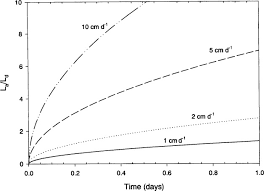The image above represents diffusion flux.

To compute for diffusion flux, three essential parameters are needed and these parameters are Mass Transfer (M), Cross-sectional Area (A) and Time (t).

The formula for calculating diffusion flux:

J = M/At

Where:

J = Diffusion Flux
M = Mass Transfer
A = Cross-sectional Area
t = Time

Given an example;
Find the diffusion flux when the mass transfer is 8, the cross-sectional area is 2 and the time is 6.

This implies that;

M = Mass Transfer = 8
A = Cross-sectional Area = 2
t = Time = 6

J = M/At
J = 8/(2)(6)
J = 8/12
J = 0.66

Therefore, the diffusion flux is 0.66 mol m-2 s-1.

## How to Calculate and Solve for Cross-Sectional Area | Electric Resistivity

The cross-sectional area is illustrated by the image below.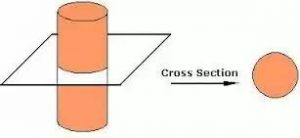To compute for cross-sectional area, three essential parameters are needed and these parameters are Resistivity (ρ), Length (l) and Resistance (R).

The formula for calculating cross-sectional area:

A = pl/R

Where:

A = Cross-sectional Area
ρ = resistivity
R = Resistance
l = Length

Let’s solve an example;
Find the cross-sectional area when the resistivity is 4, the resistance is 12 and length is 6.

This implies that;

ρ = resistivity = 4
R = Resistance = 12
l = Length = 6

A = pl/R
A = (4)(6)/12
A = (24)/12
A = 2

Therefore, the cross-sectional area is 2m².

## How to Calculate and Solve for Resistivity | Electric Resistivity

The resistivity is illustrated by the image below.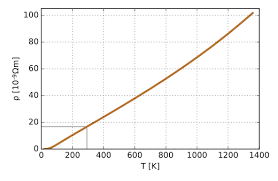To compute for resistivity, three essential parameters are needed and these parameters are Cross-sectional Area (A), Length (l) and Resistance (R).

The formula for calculating the resistivity:

ρ = RA/l

Where:
ρ = resistivity
R = Resistance
A = Cross-sectional Area
l = Length

Let’s solve an example;
Find the resistivity when the resistance is 10, the cross-sectional area is 4 and the length is 2.

This implies that;

R = Resistance = 10
A = Cross-sectional Area = 4
l = Length = 2

ρ = RA/l
ρ = (2)(10)/4
ρ = (20)/4
ρ = 5

Therefore, the resistivity is 5Ω-m.

## How to Calculate and Solve for Corrosion Penetration Rate | Corrosion

The corrosion penetration rate is illustrated by the image below.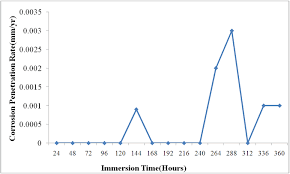To compute for corrosion penetration rate, five essential parameters are needed and these parameters are Constant (k), Weight Loss after Corrosion (W), Density of Corroding Media (ρ), Cross-sectional Area (A) and Time (t).

The formula for calculating corrosion penetration rate:

CPR = kW/ρAt

Where:

CPR = Corrosion Penetration Rate
k = Constant
W = Weight Loss after Corrosion
ρ = Density of Corroding Media
A = Cross-sectional Area
t = Time

Let’s solve an example;
Find the corrosion penetration rate when the constant is 2, the weight loss after corrosion is 4, the density of corroding media is 6, the cross-sectional area is 12 and time is 7.

This implies that;

k = Constant = 2
W = Weight Loss after Corrosion = 4
ρ = Density of Corroding Media = 6
A = Cross-sectional Area = 12
t = Time = 7

CPR = kW/ρAt
CPR = (2)(4)/(6)(12)(7)
CPR = 8/504
CPR = 0.0158

Therefore, the corrosion penetration rate is 0.0158.

## How to Calculate and Solve for Cold Crushing Strength | Refractories

The cold crushing strength is illustrated by the image below.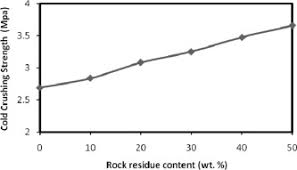To compute for cold crushing strength, two essential parameters are needed and these parameters are Maximum Load (MI) and Cross-sectional Area (A).

The formula for calculating cold crushing strength:

CCS = MI/A

Where:

CCS = Cold Crushing Strength
A = Cross-sectional Area

Let’s solve an example;
Find the cold crushing strength when the maximum load is 44 and the cross-sectional area is 11.

This implies that;

MI = Maximum Load = 44
A = Cross-sectional Area = 11

CCS = MI/A
CCS = 44/11
CCS = 4

Therefore, the cold crushing strength is 4 Pa.

Calculating the Maximum Load when the Cold Crushing Strength and the Cross-Sectional Area is Given.

MI = CCS x A

Where:

CCS = Cold Crushing Strength
A = Cross-sectional Area

Let’s solve an example;
Given that the cold crushing strength is 15 and the cross-sectional area is 5. Find the maximum load?

This implies that;

CCS = Cold Crushing Strength = 15
A = Cross-sectional Area = 5

MI = CCS x A
MI = 15 x 5
MI = 75

Therefore, the cold crushing strength is 75.

## How to Calculate and Solve for Permeability Number | Refractories

The permeability number is illustrated by the image below.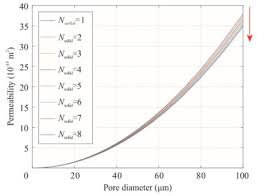To compute for the permeability number, five essential parameters are needed and these parameters are Volume of Air (V), Height of the Specimen (H), Cross-sectional Area (A), Air Pressure (P) and Time (t).

The formula for calculating permeability number:

PN = VH/APt

Where:

PN = Permeability Number
V = Volume of Air
H = Height of the Specimen
A = Cross-sectional Area
P = Air Pressure
t = Time

Let’s solve an example;
Find the permeability number when the volume of air is 2, the height of the specimen is 7, the cross-sectional area is 3, the air pressure is 9 and the time is 4.

This implies that;

V = Volume of Air = 2
H = Height of the Specimen = 7
A = Cross-sectional Area = 3
P = Air Pressure = 9
t = Time = 4

PN = VH/APt
PN = (2)(7)/(3)(9)(4)
PN = (14)/(108)
PN = 0.129

Therefore, the permeability number is 0.129.

## How to Calculate and Solve for Flow Rate | Darcy’s Law | Transport Phenomena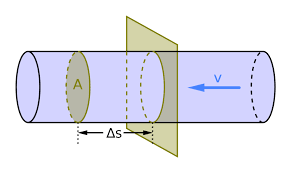The image above represents flow rate.

To compute for flow rate, four essential parameters are needed and these parameters are Permeability (K), Cross-Sectional Area (A), Change in Pressure (ΔP) and Length of Pipe (L).

The formula for calculating flow rate:

Q = KAΔP / L

Where:

Q = Flow Rate | Darcy’s Law
K = Permeability
A = Cross-sectional Area
ΔP = Change in Pressure
L = Length of Pipe

Let’s solve an example;
Find the flow rate when the permeability is 9, the cross-sectional area is 12, the change in pressure is 3 and the length of pipe is 10.

This implies that;

K = Permeability = 9
A = Cross-sectional Area = 12
ΔP = Change in Pressure = 3
L = Length of Pipe = 10

Q = KAΔP / L
Q = (9)(12)(3) / 10
Q = 324 / 10
Q = 32.4

Therefore, the flow rate is 32.4 m³/s.

Calculating the Permeability when the Flow Rate, the Cross-Sectional Area, the Change in Pressure and the Length of Pipe is Given.

K = Q x L / AΔP

Where:

K = Permeability
Q = Flow Rate | Darcy’s Law
A = Cross-sectional Area
ΔP = Change in Pressure
L = Length of Pipe

Let’s solve an example;
Find the permeability when the flow rate is 14, the cross-sectional area is 4, the change in pressure is 7 and the length of pipe is 2.

This implies that;

Q = Flow Rate | Darcy’s Law = 14
A = Cross-sectional Area = 4
ΔP = Change in Pressure = 7
L = Length of Pipe = 2

K = Q x L / AΔP
K = 14 x 2 / (4)(7)
K = 28 / 28
K = 1

Therefore, the permeability is 1.

## How to Calculate and Solve for Intensity of Induced Magnetization | Magnetic Field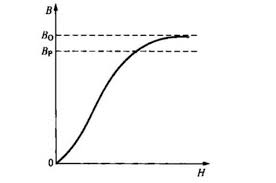The image above represents intensity induced magnetization.

To compute for intensity induced magnetization, three essential parameters are needed and these parameters are Magnetic Moment (M), Length (L) and Cross-Sectional Area (A).

The formula for calculating intensity induced magnetization:

J = M / LA

Where:

J = Intensity of Induced Magnetization
M = Magnetic Moment
L = Length
A = Cross-Sectional Area

Let’s solve an example;
Find the intensity induced magnetization when the magnetic moment is 20, the length is 16 and the cross-sectional area is 25.

This implies that;

M = Magnetic Moment = 20
L = Length = 16
A = Cross-Sectional Area = 25

J = M / LA
J = 20 / (16)(25)
J = 20 / 400
J = 0.05

Therefore, the intensity induced magnetization is 0.05.

Calculating the Magnetic Moment when the Intensity Induced Magnetization, the Length and the Cross-Sectional Area is Given.

M = J x LA

Where;

M = Magnetic Moment
J = Intensity Induced Magnetization
L = Length
A = Cross-Sectional Area

Let’s solve an example;
Find the Magnetic Moment when the intensity induced magnetization is 20, the length is 12 and the cross-sectional area is 5.

This implies that;

J = Intensity Induced Magnetization = 20
L = Length = 12
A = Cross-Sectional Area = 5

M = J x LA
M = 20 x (12)(5)
M = 20 x 60
M = 1200

Therefore, the magnetic moment is 1200.

## How to Calculate and Solve for Average Linear Velocity | Aquifer Characteristics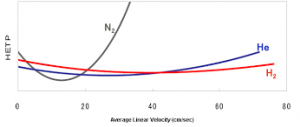The image above represents average linear velocity.

To compute for average linear velocity, three essential parameters are needed and these parameters are Discharge (Q), Effective Porosity (neand Cross-Sectional Area (A).

The formula for calculating average linear velocity:

vx = Q / neA

Where:

vx = Average Linear Velocity
Q = Discharge
ne = Effective Porosity
A = Cross-Sectional Area

Let’s solve an example;
Find the average linear velocity when the discharge is 12, the effective porosity is 9 and the cross-sectional area is 22.

This implies that;

Q = Discharge = 12
ne = Effective Porosity = 9
A = Cross-Sectional Area = 22

vx = Q / neA
vx = 12 / 9(22)
vx = 12 / 198
vx = 0.060

Therefore, the average linear velocity is 0.060 m/s.

Calculating the Discharge when the Average Linear Velocity, the Effective Porosity and the Cross-Sectional Area is Given.

Q = vx x ne A

Where;

Q = Discharge
vx = Average Linear Velocity
ne = Effective Porosity
A = Cross-Sectional Area

Let’s solve an example;
Find the discharge when the average linear velocity is 22, the effective pororsity is 10 and the cross-sectional area is 14.

This implies that;

vx = Average Linear Velocity = 22
ne = Effective Porosity = 10
A = Cross-Sectional Area = 14

Q = vx x ne A
Q = 22 x 10 x 14
Q = 3080

Therefore, the discharge is 3080.